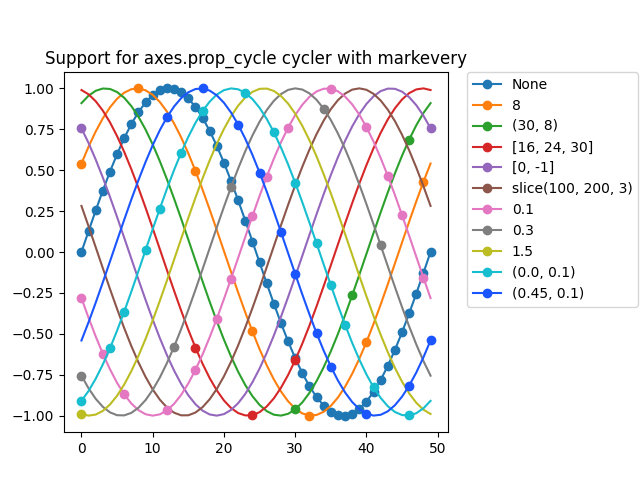# prop_cycle property markevery in rcParams#

This example demonstrates a working solution to issue #8576, providing full support of the markevery property for axes.prop_cycle assignments through rcParams. Makes use of the same list of markevery cases from the markevery demo.

Renders a plot with shifted-sine curves along each column with a unique markevery value for each sine curve.from cycler import cycler
import numpy as np
import matplotlib as mpl
import matplotlib.pyplot as plt

# Define a list of markevery cases and color cases to plot
cases = [None,
8,
(30, 8),
[16, 24, 30],
[0, -1],
slice(100, 200, 3),
0.1,
0.3,
1.5,
(0.0, 0.1),
(0.45, 0.1)]

colors = ['#1f77b4',
'#ff7f0e',
'#2ca02c',
'#d62728',
'#9467bd',
'#8c564b',
'#e377c2',
'#7f7f7f',
'#bcbd22',
'#17becf',
'#1a55FF']

# Configure rcParams axes.prop_cycle to simultaneously cycle cases and colors.
mpl.rcParams['axes.prop_cycle'] = cycler(markevery=cases, color=colors)

# Create data points and offsets
x = np.linspace(0, 2 * np.pi)
offsets = np.linspace(0, 2 * np.pi, 11, endpoint=False)
yy = np.transpose([np.sin(x + phi) for phi in offsets])

# Set the plot curve with markers and a title
fig = plt.figure()
ax = fig.add_axes([0.1, 0.1, 0.6, 0.75])

for i in range(len(cases)):
ax.plot(yy[:, i], marker='o', label=str(cases[i]))